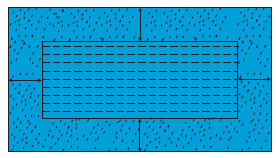#### In the centre of a rectangular lawn of dimensions 50 m × 40 m, a rectangular pond has to be constructed so that the area of the grass surrounding the pond would be 1184 m2 [see Fig. 4.1]. Find the length and breadth of the pond.length = 34m, breadth = 24m

Solution

Let the distance between the pond and loan boundary is x.

Hence the length of pond =50-x-x

From the figure

Area of lawn = Area of pond + Area of grass

50 × 40=(50-2x)(40-2x)+1184     (using Area of rectangle = l × B)

2000-1184=2000-100x-80x+4x2

4x2-180x+2000-2000+1184=0

4x2-180x+1184=0

Divide by 4

x2-45x+296=0

x2 - 37x - 8x + 296 = 0

x(x-37)-8(x-37)=0

(x-37)(x-8)=0

x = 37, 8

If x = 37

Length of pond =50-2(37)

=50-74

=-24

Which is not possible because length can’t be negative.

If x = 8

Length of pond =50-2(8)

=50-16=34m